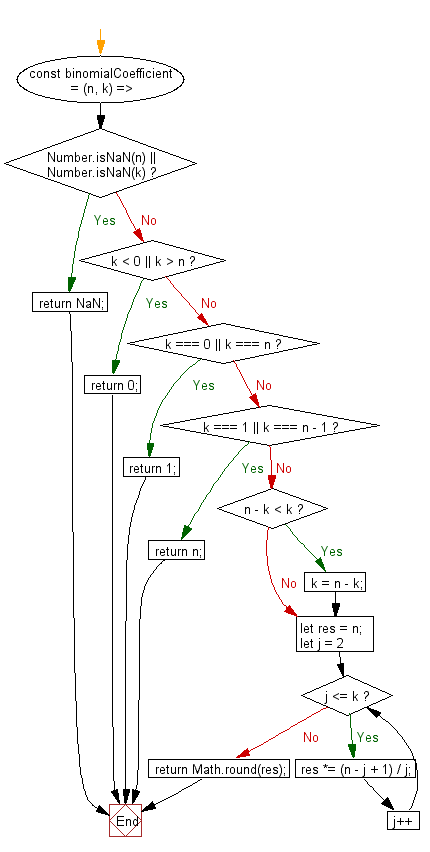# JavaScript: Evaluate the binomial coefficient of two integers n and k

## JavaScript fundamental (ES6 Syntax): Exercise-260 with Solution

Write a JavaScript program to evaluate the binomial coefficient of two integers n and k.

• Use Number.isNaN() to check if any of the two values is NaN.
• Check if k is less than 0, greater than or equal to n, equal to 1 or n - 1 and return the appropriate result.
• Check if n - k is less than k and switch their values accordingly.
• Loop from 2 through k and calculate the binomial coefficient.
• Use Math.round() to account for rounding errors in the calculation.

Sample Solution:

JavaScript Code:

``````//#Source https://bit.ly/2neWfJ2
const binomialCoefficient = (n, k) => {
if (Number.isNaN(n) || Number.isNaN(k)) return NaN;
if (k < 0 || k > n) return 0;
if (k === 0 || k === n) return 1;
if (k === 1 || k === n - 1) return n;
if (n - k < k) k = n - k;
let res = n;
for (let j = 2; j <= k; j++) res *= (n - j + 1) / j;
return Math.round(res);
};
console.log(binomialCoefficient(8, 2));
```
```

Sample Output:

```28
```

Flowchart:Live Demo:

See the Pen javascript-basic-exercise-260-1 by w3resource (@w3resource) on CodePen.

Improve this sample solution and post your code through Disqus

What is the difficulty level of this exercise?

Test your Programming skills with w3resource's quiz.

﻿

## JavaScript: Tips of the Day

How to check whether a string contains a substring in JavaScript?

ECMAScript 6 introduced String.prototype.includes:

```const string = "foo";
const substring = "oo";

console.log(string.includes(substring));
```

includes doesn't have Internet Explorer support, though. In ECMAScript 5 or older environments, use String.prototype.indexOf, which returns -1 when a substring cannot be found:

```var string = "foo";
var substring = "oo";

console.log(string.indexOf(substring) !== -1);
```

Ref: https://bit.ly/3fFFgZv